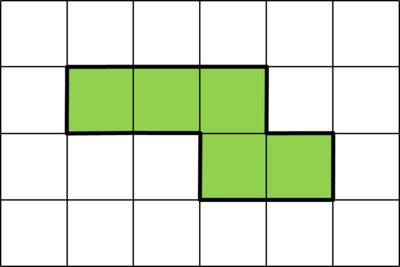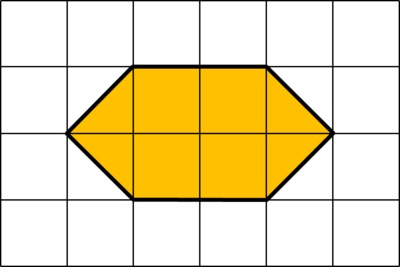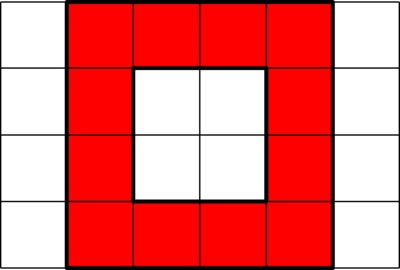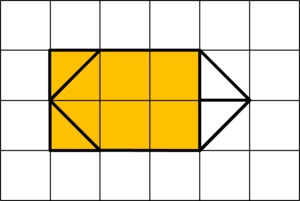# Finding the Area of Polygons

Alignments to Content Standards: 3.MD.C.6 3.MD.C.7.d

Find the area of each colored figure.

1.2.3.4.Each grid square is 1 inch long.

## IM Commentary

The purpose of this instructional task is for students to find the area of figures that can be decomposed and then recomposed into rectyangles. Students can solve parts (a) and (c) simply by using the knowledge described here:

3.MD.6 Measure areas by counting unit squares (square cm, square m, square in, square ft, and improvised units).

While students are not expected to add fractions in third grade, they can solve parts (b) and (d) by decomposing the figures and rearranging the triangles with an area of $\frac12$ square inch into whole square inches and then finding the area by counting the number of whole square inches. This strategy builds on work that students have been doing in prior grades:

K.G.6 Compose simple shapes to form larger shapes.
1.G.2 Compose two-dimensional shapes (rectangles, squares, trapezoids, triangles, half-circles, and quarter-circles) or three-dimensional shapes (cubes, right rectangular prisms, right circular cones, and right circular cylinders) to create a composite shape, and compose new shapes from the composite shape.
2.G.3 Partition circles and rectangles into two, three, or four equal shares, describe the shares using the words halves, thirds, half of, a third of, etc., and describe the whole as two halves, three thirds, four fourths. Recognize that equal shares of identical wholes need not have the same shape.

This task requires students to draw on the mathematical ideas described in the standards mentioned above and synthesize them with the mathematics described in 3.MD.7d:

3.MD.7d Recognize area as additive. Find areas of rectilinear figures by decomposing them into non-overlapping rectangles and adding the areas of the non-overlapping parts, applying this technique to solve real world problems.

While the language in these third grade standards does not explicitly describe students recomposing figures after they are decomposed in order to find the area, the fact that they have been composing figures since kindergarten suggests that such an activity should be natural for them. The Geometric Measurement Progression document makes this explicit: in third grade, "Students may first solve [their first area problems] with decomposition (cutting and/or folding) and re-composition...." Please see that document for more details http://commoncoretools.files.wordpress.com/2012/07/ccss_progression_gm_k5_2012_07_21.pdf.

While students should see tasks like this by the end of third grade because they allow students to develop connections between many mathematical ideas they have studied, these skills might be above the level of proficiency intended for grade 3 in the Common Core. This would serve as an excellent extension task.

## Solution

1. The area of the green figure is 5 square linches.
2. We can move the two triangles on the right so they match up with the two triangles on the left to form squares that are 1 inch on each side.The area of the orange figure is 6 square inches.

3. The area of the red figure is 12 square inches.
4. We can rearrange the triangles on the right so they form a rectangle.The area of the blue figure is 6 square inches.